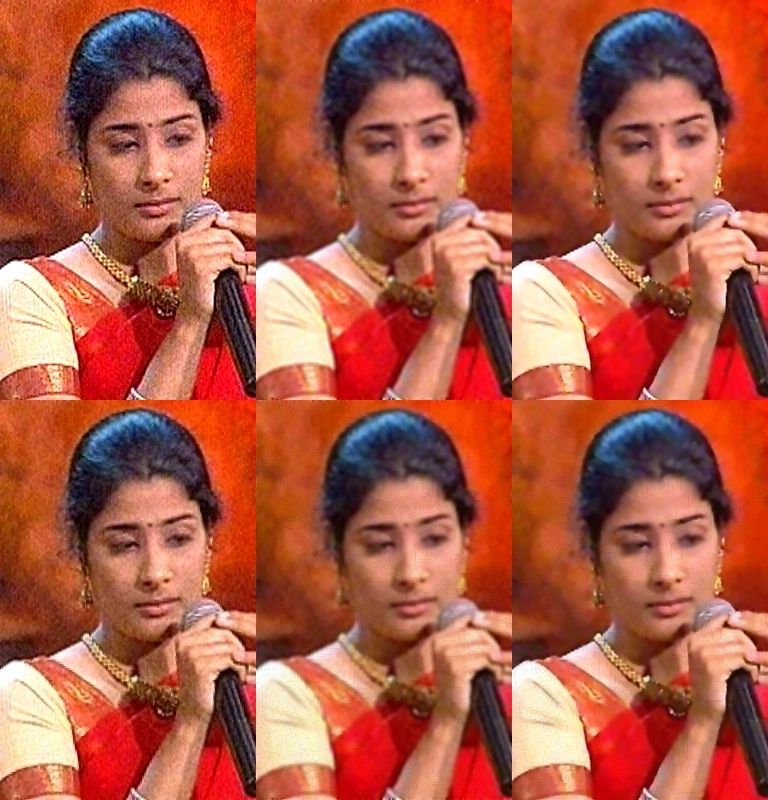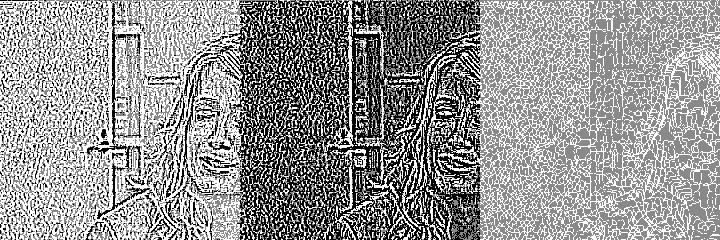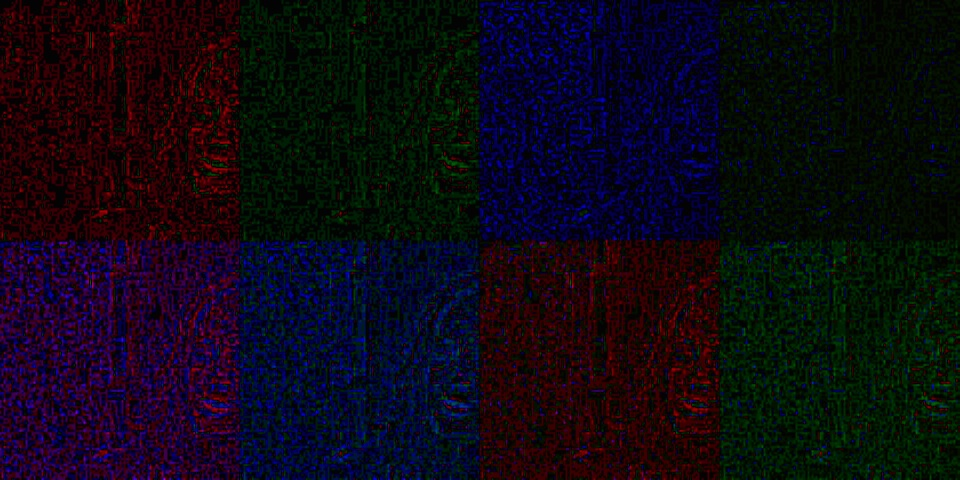SegmentedAmp

Author V. C. Mohan

Image enhancement is attempted by segmenting using Allen Soille algorithm for watershed and basin delineation. In general watershed technique over-segments. To minimise this, the input may be preprocessed (denoised using DeNoise plugin and or blurred with function GaussBlur available in ColorIt plugin or numerous other functions available for Avisynth) to reduce segmentation.

This plugin smoothens within segments (basins) and or accentuates (sharpens) the segment boundaries to visually enhance the image. For RGB or Y plane of YUY2 or YV12 formats. sm is smoothening factor with values between 0 and 10. Sh is sharpening factor with value between -10 to 10. Both should not be simultaneously zero. sh values of -2 or so appears to yield better results.

RGB32, RGB24, YUY2 and YV12 formats are supported. Smoothenig can be done on u, v planes of YUY2 and Planar (YV12 only in 2.5 version) Sharpening is always limited to Y values.

Segmention can be done on the image of a different clip (sclip) and used for smoothing or sharpening. For instance, a blurred clip or denoised clip can be used as the sclip.

This function runs slow and needs optimization to speed up

 Description Name Type Limits Default Input clip clip none sharpening factor for RGB or Y values sh integer -10 to 10, both sh and sm must not be 0 for RGB formats -2 smoothing factor for RGB or Y plane sm integer 0 to 10, both sh and sm must not be 0 for RGB formats 2 smoothing factor for U plane(for YUY and YV12 only) smu integer 0 to 10 2 smoothing factor for v plane(for YUY and YV12 only) smv integer 0 to 10 2 nearest 4 or 8 to be used for connectivity c4 boolean true for connect 4, false for connect 8 true Use a different clip for segmenting? useclip bool true for use clip. false for segment and process same clip false clip for segmenting if useclip is true sclip clip valid clip. Not the one at first parameter need not be specified if useclip is false

#Usage examples:-

dn = denoise(......)
segmentedamp(dn, useclip = false,sh = 0, sm = 10 )
segmentedamp(img, useclip = false,  sh = -5, sm = 0 )
inv1 = invert(dn)
segmentedamp(img, useclip = true, sclip = inv1, sh =-1,sm = 10)
gb = gaussblur(img,size=5, sd = 3.5)
segmentedamp(dn, useclip = true,sclip = gb, sh =-5, sm =4)

below are illustration of results. Top row are inputs. Left is the original image. Center is Gaussian blurred image. Right is DeNoised image. Bottom row is the output using this plugin. script used for this output is:-

sh0 =segmentedamp(gb, useclip = false, sh = -6, sm = 10, smu = 0, smv = 0 )
sh1=segmentedamp(dn, useclip = false,  sh = -6, sm = 2 , smu = 0, smv = 0)
sh2=segmentedamp(img, useclip = false, sh =0,sm = 10)
s1 = stackhorizontal(img,gb,dn)
s2 = stackhorizontal(sh2,sh0, sh1)
stackvertical(s1,s2)# script used for following output

smy=segmentedAmp(imgl, sm = 1, sh = 0 )
smyi=segmentedAmp(imgl, sm = 10, sh = 0)
shy=segmentedAmp(imgl,sm = 0, sh = -1 )
submy = subtract(imgl, smy)
submyi = subtract(imgl, smyi)
subhy = subtract(imgl, shy)
stackhorizontal(submy.crop(300,0,240,240),submyi.crop(300,0,240,240),subhy.crop(300,0,240,240)).Levels(127,1,129,0,255)

Left top diff without inversion, middle with inversion, right shows individual cells# This example indicates the noise that can be removed with various combinations
of u, v planes smoothing using prior inversions. Y plane not processed
iv = invert(img1)
sv=segmentedAmp(imgl, smv = 8)
svi=segmentedAmp(imgl,useclip = true, sclip = iv, smv = 8)
su=segmentedAmp(imgl, smu = 8)
sui=segmentedAmp(imgl,useclip = true, sclip = iv, smu = 8)

suv=segmentedAmp(imgl, yy =false,uu = true,smU = 8, vv=true,iu = false,iv = false, smv = 8)
suvi=segmentedAmp(imgl, useclip = true, sclip = iv, smv = 8)
suiv=segmentedAmp(imgl, useclip = true, sclip = iv, smu = 8,smv = 8)
suivi=segmentedAmp(imgl,useclip = true, sclip = iv, smu = 8,smv = 8)

sub1 = subtract ( imgl,sv)# v only. no inversion
sub2 = subtract ( imgl,svi)# inverted v only
sub3 = subtract ( imgl,su) * u only
sub4 = subtract ( imgl,sui)# inverted u only

sub5 = subtract ( imgl,suv) # u, v both normal
sub6 = subtract ( imgl,suvi)# u normal, v inverted
sub7 = subtract ( imgl,suiv)# inverted u, normal v
sub8 = subtract ( imgl,suivi)# both u, v inverted

st1 = stackhorizontal(sub1.crop(300,0,240,240),sub2.crop(300,0,240,240),sub3.crop(300,0,240,240), sub4.crop(300,0,240,240)).Levels(128,1,135,0,255)
st2 = stackhorizontal(sub5.crop(300,0,240,240),sub6.crop(300,0,240,240),sub7.crop(300,0,240,240), sub8.crop(300,0,240,240)).Levels(128,1,135,0,255)
stackvertical(st1,st2)

Panels show noise removed
Top from left: v only, inverted v only, u only, inverted u only
Bottom from left : u&v, u & inverted v, inverted u & v, both u & v invertedTo my index page To Avisynth